# Power of cosine window

The power of cosine window coefficients are given by

$$a(k)=\cos^\alpha (\frac{\pi k}{N-1}-\frac{\pi}{2})$$

where N is the length of the filter, k = 0, 1, …, N – 1, and α > 0.

At α = 0, the formula above produces the rectangular window. The sine window is the power of cosine window with α = 1. At α = 2, the power of cosine window becomes the Hann window by the standard double angle trigonometric identity.

Take a finite impulse response (FIR) low pass filter of length N = 201. The following is the power of cosine window for α = 3.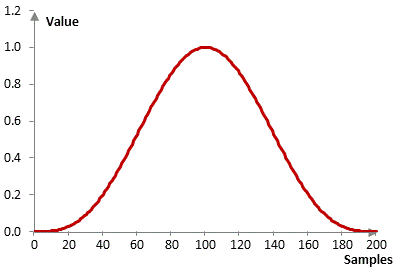Given a sampling frequency of 2000 Hz and a filter cutoff frequency of 40 Hz, the impulse response of the filter with a rectangular window (with no window) and with the power of cosine window is as follows.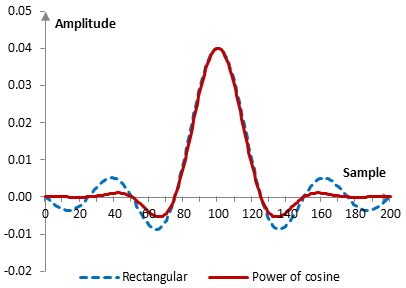The magnitude response of the same filter is shown on the graph below.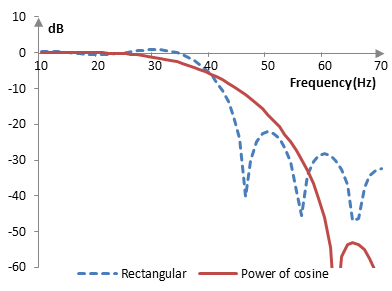With larger α, the window further depresses the side lobes of the impulse response of the window and the corresponding filter produces better stop band attenuation at the expense of a larger transition band. The following is the power of cosine window at three different values of α (1, 2, and 3).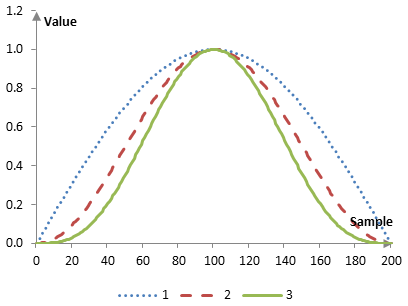The magnitude responses of the corresponding filters are as follows.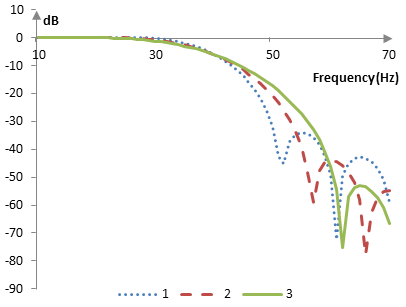## Measures for the power of cosine window

The following is a comparison of the discrete Fourier transform of the power of cosine window (α = 3) and the rectangular window.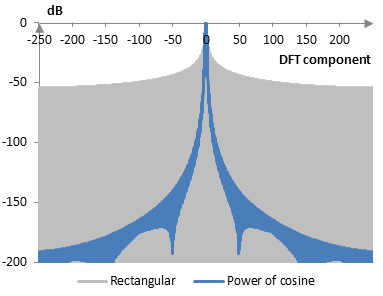The power of cosine window measures are as follows.

 α 1.0 2.0 3.0 Coherent gain 0.64 0.50 0.42 Equivalent noise bandwidth 1.24 1.50 1.74 Processing gain -0.92 dB -1.77 dB -2.40 dB Scalloping loss -2.09 dB -1.42 dB -1.07 dB Worst case processing loss -3.01 dB -3.19 dB -3.47 dB Highest sidelobe level -23.0 dB -31.5 dB -39.3 dB Sidelobe falloff -11.2 dB / octave, -37.1 dB / decade -20.7 dB / octave, -68.9 dB / decade -21.3 dB / octave, -70.6 dB / decade Main lobe is -3 dB 1.18 bins 1.44 bins 1.66 bins Main lobe is -6 dB 1.64 bins 2.00 bins 2.32 bins Overlap correlation at 50% overlap 0.317 0.165 0.084 Amplitude flatness at 50% overlap 0.707 1.000 0.707 Overlap correlation at 75% overlap 0.755 0.568 0.565 Amplitude flatness at 75% overlap 0.924 1.000 0.990

Window

### Filtered HTML

• Freelinking helps you easily create HTML links. Links take the form of [[indicator:target|Title]]. By default (no indicator): Click to view a local node.